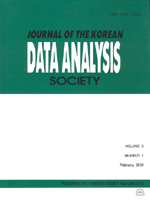상세검색
최근 검색어 전체 삭제
다국어입력
즐겨찾기0KCI등재 학술저널

# Dimension Reduction and Prediction for High-dimensional Regression Models Using the Graphical Lasso

• 등재여부 : KCI등재
• 2013.10
• 2321 - 2332 (12 pages)
• 7

Deriving reductions from high-dimensional covariates with minimal loss of information is an effective way of reducing covariate dimension and plays a pivotal role in sufficient dimension reduction (SDR). We investigate sufficient dimension reduction in highdimensional regressions by using the graphical lasso method developed by Friedman, Hastie, Tibshirani (2007) even when the sample size n is not sufficiently large compared to the number of predictors p. The key point here is that we consider the model in abundant highdimensional regressions where most of the covariates contribute some information on a response, which differ from sparse regressions. By discovering an intrinsic dimensionality of the data and estimating a sufficient reduction R(X), we improve the prediction of the response, which is our ultimate goal, with a kernel-type estimator. The estimated reductions and predicted values of the response are illustrated through simulation studies and a real data application.

1. Introduction

2. Estimation

3. Graphical lasso

4. Simulation study

5. Real data application

6. Concluding Remarks

References